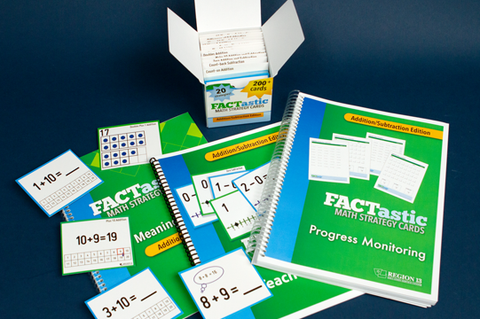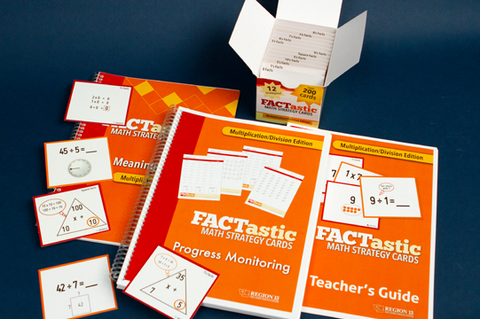# FACTastic Math Strategy System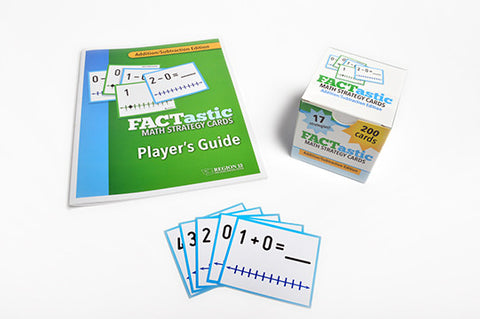FACTastic Math Strategy System: Addition/Subtraction (Set of Cards and Spiral-Bound Teacher's Guide)

\$ 45.00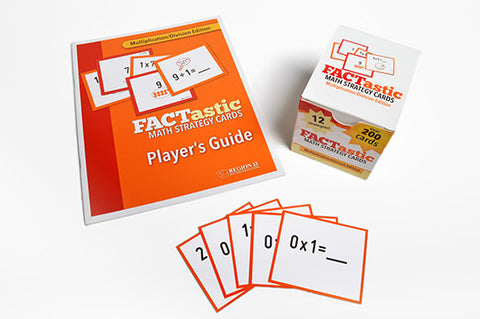FACTastic Math Strategy System: Multiplication/Division (Set of Cards and Spiral-Bound Teacher's Guide)

\$ 45.00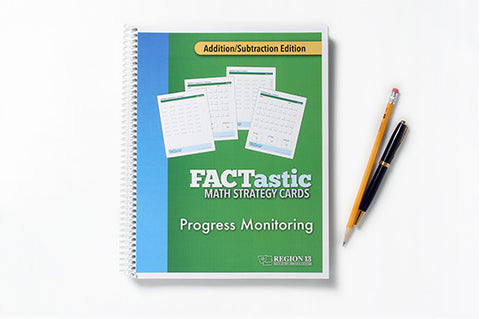FACTastic Math Progress Monitoring: Addition/Subtraction (Spiral-Bound)

\$ 20.00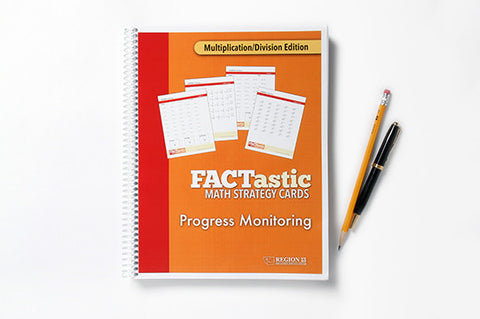FACTastic Math Progress Monitoring: Multiplication/Division (Spiral-Bound)

\$ 20.00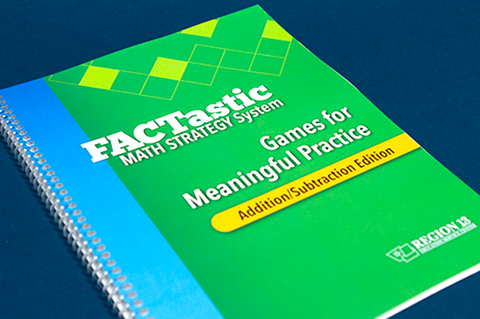FACTastic Math Strategy System Games for Meaningful Practice: Addition/Subtraction (Spiral-Bound)

\$ 30.00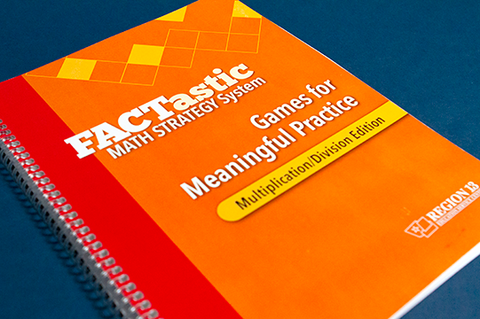FACTastic Math Strategy System Games for Meaningful Practice: Multiplication/Division (Spiral-Bound)

\$ 30.00